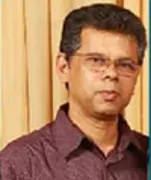X
Numerical estimation of nonlinear wave forces on a multi-hull barge using finite element method
Aswathy Senan,
Published in
2012
Volume: 5

Pages: 35 - 42
Abstract
This paper deals with the estimation of second order wave excitation forces on a free floating triple-hull barge using software developed based on finite element method. The wave-hull interaction nonlinear problem is presented here using the perturbation method, where potential flow theory is used. In the finite element model, the absorbing or nonreflecting far boundary condition is applied at a truncated surface in the form of boundary damper. The software developed for the solution of this nonlinear problem is validated for two and three-dimensional cases for which analytical and other numerical solutions are known. A convergence study on the three-dimensional cylinder problem is carried out to derive a guideline in selecting finite element mesh density and its grading. A triple-hull barge problem is selected here as a practical problem to study the nonlinear wave effects on the forces and motions. A grid independent study on this problem is carried out by using four finite element meshes of different density and grading. The optimum mesh selected from this study is used for further analysis of the problem. Bandwidth optimization is carried out on the generated meshes in order to reduce the computational effort, as the finite element algorithm used here is based on the banded solver technique. The second order wave excitation forces and moments on the barge estimated for different wave steepness (H/Lw - 0.08 to 0.012) in oblique sea condition shows that the second order surge and heave forces amounts up to 49% and 17.5% respectively and the second order yaw moment up to 39% when compared with the first order (linear) wave forces. Similar trend is observed for the forces and moments in beam sea and head sea conditions. Copyright © 2012 by ASME.
Journal Proceedings of the International Conference on Offshore Mechanics and Arctic Engineering - OMAE No
Authors (1)
•Concepts (15)
•BANDWIDTH OPTIMIZATION
•FAR-BOUNDARY CONDITION
•FINITE ELEMENT ALGORITHMS
•FINITE ELEMENT MESHES
•NON-LINEAR WAVE FORCES
•POTENTIAL-FLOW THEORY
•SECOND ORDER WAVES
•Wave-structure interaction
•ARCTIC ENGINEERING
•Finite element method
•••••